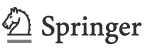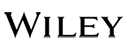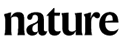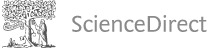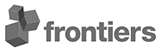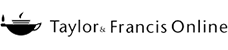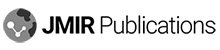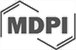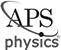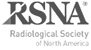# Covariance Calculator

Use this calculator to estimate the covariance of any two sets of data. It computes the sample covariance and population covariance of two variables. The calculator supports weighted covariance and also outputs the sample means.

Share calculator:

Embed this tool:
get code

## What is covariance?

In statistics, the phenomenon measured by covariance is that of statistical correlation. We say two random variables or bivariate data vary together if there is some form of quantifiable association between them. A trivial example is the change in the intensity of cloud coverage and rainfall precipitation in a given region. Plotting the two variables, we will observe that they tend to change together, suggesting some statistical dependence between them. Such joint variability can be due to direct causality, indirect causality, or entirely spurious.

Covariance works under the assumption of linear dependence. The sign of the covariance calculated for two variables, X and Y, (denoted cov(X,Y)) shows the direction in which the dependent variable (Y) tends to change with changes in the independent variable (X). A positive covariance means that increasing values of X are associated with increasing values in Y. Negative covariance shows an inverse relationship: increasing values in X are associated with decreasing values in Y.

A covariance of zero signifies complete lack of a statistical association (orthogonality), but not necessarily statistical independence. For other values of cov(X,Y) the magnitude is difficult to interpret in practice as it depends on the scale of the values of both variables. This is the reason why for most practical purposes a standardized version of covariance called a correlation coefficient is used instead. It makes comparisons of the joint variability between variables on different scales possible.

## Using the covariance calculator

To use the calculator, first enter the data you want to analyze: one column per variable, X and Y. Optionally, you can enter pair weights in a third column, in which case they will be applied to the values resulting in a weighted covariance. Columns need to be separated by spaces, tabs, or commas. Copy-pasting from Excel or another spreadsheet software should work just fine. All columns should have an equal number of rows in them.

When you press 'Calculate' the covariance calculator will produce as output the sample covariance, population covariance (see below for the differences between the two), the arithmetic mean of X, the mean of Y, and the count of samples (pairs).

## Covariance formula

There are two slightly different equations for calculating covariance. Which one is applicable depends on the particular type of data and analysis, as explained below.

### Population covariance formula

The formula for computing population covariance is: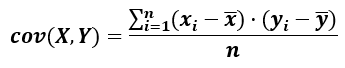where cov(X,Y) means the covariance of the variables X and Y and Σ is the Greek upper-case letter "sigma", the commonly used symbol for mathematical summation, x-bar is the sample mean of the X data set (x-mean), y-bar is the sample mean of the Y data set, and xi and yi are elements of these datasets indexed by i. n is simply the number of elements in each data set. This formula is applicable if the observed values of X and Y consist of the entire population of interest and in such case it is a population parameter stemming from the joint probability distribution. As this is rare in practice, to calculate covariance one most often uses the covariance formula below.

### Sample covariance formula

The formula for sample covariance is: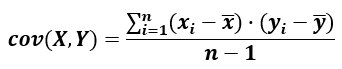which is essentially the same as for population covariance, but the denominator is n-1 instead of just n. This adjustment reflects the additional degree of freedom that comes from the data being just a sample. Such a covariance is a statistical estimate of the covariance of a larger population based on samples from two random variables.

Both equations are supported by our covariance calculator so it is great way to easily explore the relationship between the two estimates.

## Applications of covariance

Covariance has applications in multiple scientific and applied disciplines such as financial economics, genetics, molecular biology, machine learning, and others. Covariance matrices are used in principle component analysis (PCA) which reduces feature dimensionality in data preprocessing.

Calculating covariance is a step in the calculation of a correlation coefficient. A covariance matrix is the basis of a correlation matrix. Normally correlation coefficients are preferred due to their standardized measure which makes it easy to compare covariances across many differently scaled variables.

## Practical example

In this example we will settle for the simpler problem of the association between smoking and life duration. What would the joint variability of these two variables look like for a given research sample? Let's say we take a representative sample of fifteen men fifty years and older who smoke, and measure both the number of cigarettes they consume per day and the age at which they died. The number of cigarettes is the independent variable X, whereas life duration in years is the dependent variable Y.

Example data for examining covariance
Measure / Case010203040506070809101112131415
Cigarettes/day 25 46 17 26 5 23 24 35 29 4 13 8 6 23 19
Lifespan (years) 60 53 86 77 78 77 65 72 58 91 66 84 73 78 75

By using the calculator we get a resulting sample covariance of -85.90. The negative sign suggests an inverse relationship between smoking and longevity - the more cigarettes per day, the shorter the lifespan.

Here is how the scatterplot of the two variables looks like: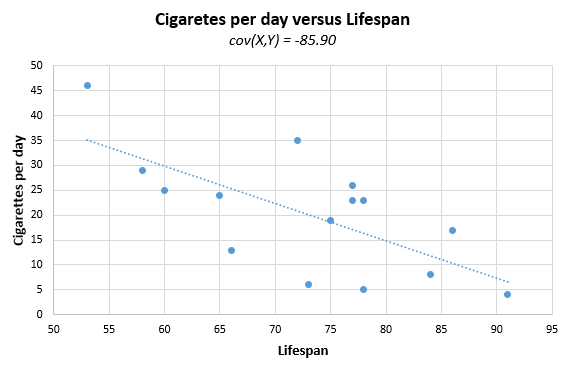Note the slope is descending which is characteristic of negative covariance. If the covariance was positive, the slope would be ascending. If there was no association between the two, the slope would be zero degrees.

#### Cite this calculator & page

If you'd like to cite this online calculator resource and information as provided on the page, you can use the following citation:
Georgiev G.Z., "Covariance Calculator", [online] Available at: https://www.gigacalculator.com/calculators/covariance-calculator.php URL [Accessed Date: 07 Jul, 2022].

Our statistical calculators have been featured in scientific papers and articles published in high-profile science journals by: